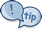# Mathway’s Free Math SolverRefresh your browser if it doesn’t load the first time. Trust me, it happens.

## Mathway

I must say that there is no alternative to paying attention in class, writing down notes, studying at home, and doing a lot of independent practice using pencil and paper. However, there are times when a teacher or tutor is not available to help you solve a math problem. This can be frustrating at times. But, don’t worry. Mathway can be that extra “eye” to help double-check your work. Whether it’s math homework in pre-algebra, algebra, geometry, trigonometry, precalculus, statistics, or calculus, this is the tool for you. Heck, you can even just use it as a regular arithmetic calculator! Moreover, you can also use this as a graphing utility to graph any functions such as linear, quadratic, trigonometric functions, etc. To be honest, I start wondering what this math solver cannot do!

Mathway’s math problem solver is an excellent tool to check your work for free. Just type in the math problem into the interactive calculator using its prescribed syntax, and it will give you the correct answer. It’s like having a private tutor 24/7/365 with you at all times!

Note: If you want more help, and therefore, would want to see how Mathway solves the problem step by step, you can click “Tap to view steps…” to redirect you to the developer’s website for a modest subscription fee which in return, I will receive some monetary compensation.

However, if what you need is to simply check if you arrived at the correct answer and not necessarily detailed step-by-step answers, then the widget should be sufficient for you.

Similar Solver:

Unit Conversion Calculator